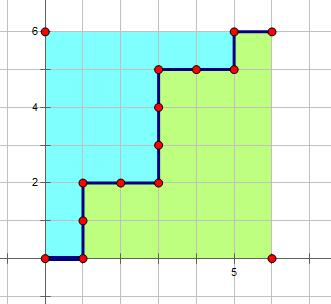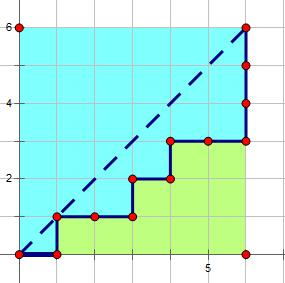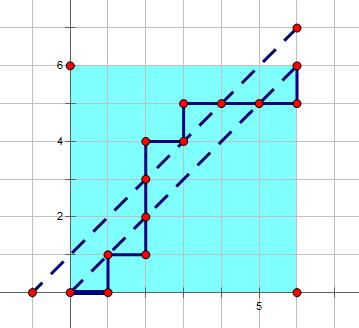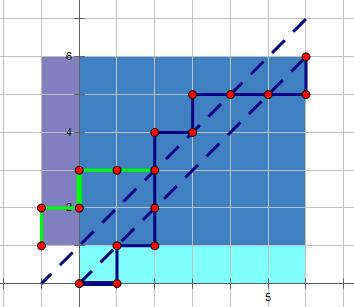# 一个典型的路径统计问题

## 简单版的问题## 复杂一点$$\binom{2n}{n}-\binom{2n}{n+1}$$

# 卡特兰数的不同形态

## 封闭形式

$$C_{n}=\binom{2n}{n}-\binom{2n}{n+1},n>0$$

$$C_{n}=\frac{\binom{2n}{n}}{n+1},n>0$$

## 卷积形式

$$C_{n}=\sum_{k}C_kC_{n-1-k}+[n=0],n>0$$

## 递推形式

$$C_0=1,C_{n+1}=\frac{2(2n+1)}{n+2}C_n$$

# 一些例子

()(())

## 凸多边形的三角剖分数1 3 4 5 6
2 7 8 9 10